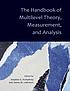# Classify

## Summary

Title:
The handbook of multilevel theory, measurement, and analysis
Author:
Humphrey, Stephen E. (Stephen Earle)
Editor:
LeBreton, James M.; Humphrey, Stephen E.
Formats:

Editions:
16
Total Holdings:
398
OCLC Work Id:
5447040641
http://classify.oclc.org/classify2/ClassifyDemo?owi=5447040641
Most Frequent 302.01 388 Web Dewey
Edition: 23 302.01 353 Web Dewey
Most Frequent HM1106 373 ClassWeb## Editions

Displaying 1 to 16 of 16
Title and AuthorLanguageFormatHoldings TagClass NumberThe handbook of multilevel theory, measurement, and analysiseng 247050HM1106
082302.01

(DDC 23)The handbook of multilevel theory, measurement, and analysiseng 73082302.01

(DDC 23)

050HM1106The handbook of multilevel theory, measurement, and analysiseng 24050HM1106
082302.01The handbook of multilevel theory, measurement, and analysiseng 17050HM1106
082302.01

(DDC 23)The Handbook of Multilevel Theory, Measurement, and Analysisby Humphrey, Stephen E. (Stephen Earle)eng 11082302.01The handbook of multilevel theory, measurement, and analysiseng 7082302.01

(DDC 23)

050HM1106The handbook of multilevel theory, measurement, and analysiseng 4082001.422

(DDC 23)The handbook of multilevel theory, measurement, and analysiseng 4082302.01

(DDC 23)The handbook of multilevel theory, measurement, and analysiseng 3082302.01

(DDC 23)

050HM1106The handbook of multilevel theory, measurement, and analysiseng 2Handbook of multilevel theory, measurement, and anlysiseng 1The handbook of multilevel theory, measurement, and analysiseng 1The handbook of multilevel theory, measurement, and analysiseng 1The handbook of multilevel theory, measurement, and analysiseng 1082302.015195

(DDC 23)The handbook of multilevel theory, measurement, and analysiseng 1082302.01

(DDC 23)

050HM1106The handbook of multilevel theory, measurement, and analysiseng 1050HM1106
082302.01

(DDC 23)

Displaying 1 to 16 of 16
.